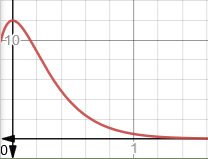## Sunday, June 20, 2021

### Viscosity Damping 2: UNIZOR.COM - Physics4Teens - Waves - Mechanical Osc...

Notes to a video lecture on http://www.unizor.com

Viscosity Damping 2
Critical Damping

This lecture continues analyzing the damped oscillation in a case of critical damping. It relies on equations and symbols presented in the previous lecture.

The main differential equation that describes the damped oscillation is
m·x"(t) + c·x'(t) + k·x(t) = 0
where
m is a mass of a object attached to a free end of a spring,
c is viscosity of environment surrounding a spring, as it acts against motion of an object,
k is elasticity of a spring,
x(t) is displacement of an object from its neutral position on a spring.
We also consider initial conditions
x(0) = a (initial stretch),
x'(0) = 0 (no initial speed).

In search of two partial solutions of the equation above we used in a previous lecture a function
x(t) = eγ·t
where γ - some (generally, complex) number.
The reason for this is that, substituting this function with its first and second derivatives into the differential equation, we get
x'(t) = γ·eγ·t
x"(t) = γ²·eγ·t
and the differential equation is
γ²·m·eγ·t + γ·c·eγ·t + k·eγ·t = 0

Canceling eγ·t, which never equal to zero, we get a quadratic equation for γ
γ²·m + γ·c + k = 0
with any of its solutions (generally, complex), used in function eγ·t, delivering the solution of the original differential equation.

Case 2
Δ=c²−4k·m EQUALS to ZERO

So, we assume now that the discriminant of the quadratic equation above is zero:
Δ = c²−4k·m = 0.
Then two roots to the quadratic equation above coincide:
γ1,2 = −c/(2m)

As we see, in this case our approach delivered only one partial solution to our differential equation:
x1(t) = e−c·t/(2m)
But, to get to a general solution, we need two partial ones.

The second partial solution we will search among functions x2(t)=t·eγ·t.
x'2(t) = eγ·t + t·γ·eγ·t
x"2(t) = γ·eγ·t + γ·eγ·t + t·γ²·eγ·t
and the differential equation is
m·[γ·eγ·t + γ·eγ·t + t·γ²·eγ·t] +
+ c·[eγ·t + t·γ·eγ·t] + k·t·eγ·t = 0

Canceling exponent eγ·t that never equals to zero, we obtain
m·[γ + γ + t·γ²] +
+ c·[1 + t·γ] + k·t = 0

Let's group the terms of this equation
t·[m·γ² + c·γ + k] + 2m·γ + c = 0

If we can find such γ that the expression above is always zero for any t, the corresponding function x2(t)=t·eγ·t will be a second partial solution we are looking for.

First of all, we should use the fact that the case we are considering now is
Δ = c²−4k·m = 0.
From this follows that
k = c²/(4m)
Substituting this into the above equation, we get
t·[m·γ² + c·γ + c²/(4m)] +
+ 2m·γ + c = 0

Now let's choose such γ that nullifies the part not involved in multiplication by t:
2m·γ + c = 0
γ = −c/(2m)
What it does with a coefficient at t is as follows:
m·γ² + c·γ + c²/(4m) =
= m·c²/(4m²) − c²/(2m) +
+ c²/(4m) =
= c²/(4m) − c²/(2m) +
+ c²/(4m) = 0

So, the coefficient at t is also equals to zero.

Therefore, with γ=−c/(2m) the quadratic equation above is satisfied for any t and function x2(t)=t·e−c·t/(2m) is the second partial solution to our differential equations.

Now, having two partial solutions
x1(t) = e−c·t/(2m) and
x2(t) = t·e−c·t/(2m),
we can formulate a general solution to critically damped oscillation, when Δ=c²−4k·m=0:
x(t) = A·x1(t) + B·x2(t) =
= A·e−c·t/(2m) + B·t·e−c·t/(2m)

We can determine coefficients A and B from initial conditions
x(0) = a and
x'(0) = 0
From this we get
A = a and
−A·c/(2m) + B = 0

Therefore, our two coefficients are A=a and B=a·c/(2m).
General solution for of a case of critical damping, when c²=4m·k, is
x(t) = a·e−c·t/(2m) +
+ (a·c/(2m))·t·e−c·t/(2m) =
= a·(1+c·t/(2m))·e−c·t/(2m)

Let's calculate the exact expression for this function for some sample values of the constants involved:
a=12
m=1
c=10
k=25
Δ=c²−4m·k=0
x(t) = 12·(1+5t)·e−5t

Now let's check if this solution satisfies our differential equation
m·x"(t) + c·x'(t) + k·x(t) = 0
Substituting the assumed values for coefficients, it looks like this:
x"(t) + 10·x'(t) + 25·x(t) = 0

Let's check it out for our sample function
x(t) = 12·(1+5t)·e−5t
x'(t) = 12·5·e−5t +
+ 12·(1+5t)·(−5)·e−5t =
= −300t·e−5t

x"(t) = −300·e−5t+1500t·e−5t

Substituting these expressions into equation
x"(t) + 10·x'(t) + 25·x(t) =
= −300·e−5t+1500t·e−5t
− 10·300t·e−5t +
+ 25·12·(1+5t)·e−5t =
= −300·e−5t + 1500t·e−5t
− 3000t·e−5t +
+ 300·e−5t + 1500t·e−5t =
= 0
(OK)

Let's check the initial conditions.
x(0)=12=a (OK)
x'(0)=0 (OK)

Let's draw a graph of our sample function
x(t) = 12·(1+5t)·e−5tThis graph confirms that the displacement of an object from the neutral position monotonically goes to zero, that is it moves in one direction only towards the neutral position without oscillation.
The effect of damping of viscous environment is so strong that it prevents the object to oscillate on a spring.
This was a case of critical damping.